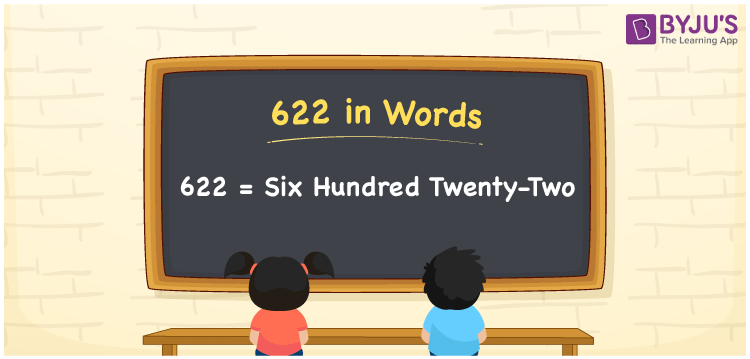# 622 in words

622 in words is written as Six Hundred and Twenty Two. 622 represents the count or value. The article on Counting Numbers can give you an idea about count or counting. The number 622 is used in expressions that relate to money, distance, length, year and others. Let us consider an example for 622. ”I need to deposit Six Hundred and Twenty Two rupees in the bank.”

 622 in words Six Hundred and Twenty Two Six Hundred and Twenty Two in Numbers 622

## 622 in English Words## How to Write 622 in Words?

We can convert 622 to words using a place value chart. The number 622 has 3 digits, so let’s make a chart that shows the place value up to 3 digits.

 Hundreds Tens Ones 6 2 2

Thus, we can write the expanded form as:

= 6 × Hundred + 2 × Ten + 2 × One

= 6 × 100 + 2 × 10 + 2 × 1

= 622

= Six Hundred and Twenty Two.

622 is the natural number that is succeeded by 621 and preceded by 623.

622 in words – Six Hundred and Twenty Two.

Is 622 an odd number? – No.

Is 622 an even number? – Yes.

Is 622 a perfect square number? – No.

Is 622 a perfect cube number? – No.

Is 622 a prime number? – No.

Is 622 a composite number? – Yes.

## Solved Example

1. Write the number 622 in expanded form

Solution: 6 × 100 + 2 × 10 + 2 × 1

We can write 622 = 600 + 20 + 2

= 6 × 100 + 2 × 10 + 2 × 1

## Frequently Asked Questions on 622 in words

Q1

### How to write 622 in words?

622 in words is written as Six Hundred and Twenty Two.
Q2

### Is 622 a perfect square number?

No. 622 is not a perfect square number.
Q3

### Is 622 a prime number?

No. 622 is not a prime number.